Temperature and strain change the grating period as well as the grating refractive
index. Consequently, the response of the grating device is changed when
temperature and strain distributions change.

## Strain-optic effect of fiber Bragg grating

The changes of optical indicatrix caused by strain are: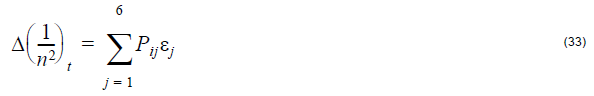where, ε1 = ε2 = –vε, ε3 = ε, ε4 = ε5 = ε6 = 0 (no shear strain), and ε
being the axial strain in the optical fiber. The symbol v denotes the Poisson’s ratio
for the fiber.

The strain-optical tensor for a homogeneous isotropic material is: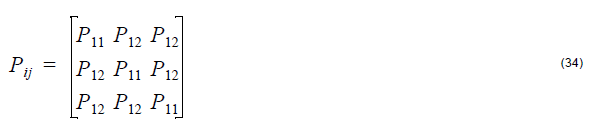where Pij are the strain-optic constants,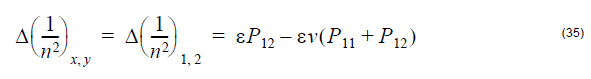The refractive index change is: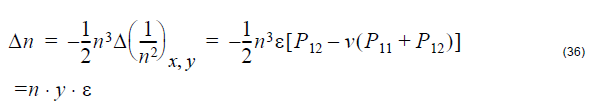where the strain-optic coefficient y is defined as: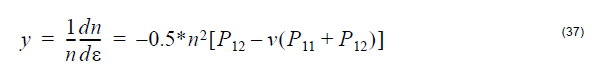The grating period changes is: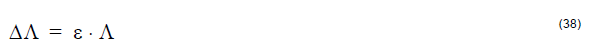The default strain distributions that can be applied to a fiber grating are listed below:

• Uniform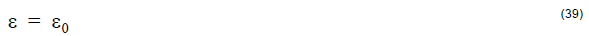where ε0 is the constant strain.

• Linear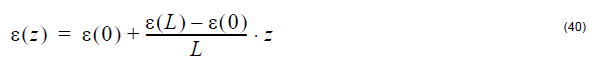where L is the grating length, ε(0) is the strain at z = 0, and ε(L) is the strain
at z =L

• Gaussian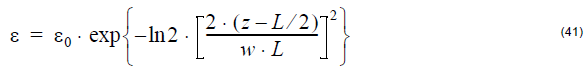where ε0 is the peak strain value and w is the normalized value of FWHM.
Other strain distributions can be defined by user functions.

## Thermal-optic effect of fiber Bragg grating

The temperature-induced refractive index change is: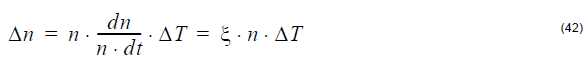where ξ is the thermo-optic coefficient of the fiber and ΔT is the temperature
change.

The temperature-induced grating period change is: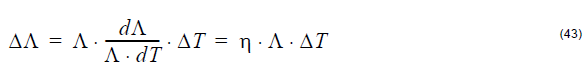where η is the thermo-optic expansion coefficient.

The default temperature distributions that can be applied to a fiber are listed below:

• Uniform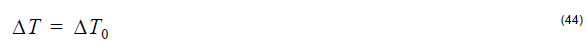where ΔT0 is the constant temperature.

• Linear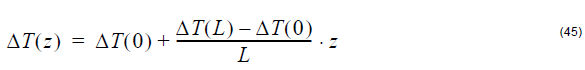where L is the grating length, ΔT(0) is the temperature at Z = 0, and ΔT(L) is
the temperature at z = L.

• Gaussian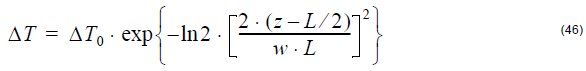where ΔT0 is the peak temperature value and w is the normalized value of FWHM.
Other temperature distributions can be defined by user functions.